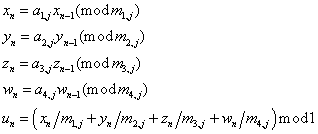ID 772987
Date 12/04/2020
Public

## WH

This is a set of 273 Wichmann-Hill’s combined multiplicative congruential generators (j = 1, 2, ..., 273):WH is a set of 273 different basic generators. This generator is the second basic generator in the NAG libraries. The constants ai,j  range from 112 to 127, the constants mi,j are prime numbers ranging from 16,718,909 to 16,776,971, close to 224. These constants should show good results in the spectral test (see Knuth [Knuth81] and MacLaren [MacLaren89]). The period of each Wichmann-Hill generator may be equal to 292 if not for common factors between (m1,j-1), (m2,j-1), (m3,j-1) and (m4,j-1). However, each generator should still have a period of at least 280. The generated pseudo-random sequences are essentially independent of one another according to the spectral test (for detailed information about properties of these generators see [MacLaren89]).

### Real Implementation (Single and Double Precision)

The output vector is the sequence of the floating-point values u0, u1, ...

### Integer Implementation

The output vector of 32-bit integers x0, y0, z0, w0, x1, y1, z1, w1

Thus, the output vector stores practically every quadruple (x, y, z, w) of members of the integer output as four 32-bit integers. For example, to get a vector from n quadruples (x, y, z, w), the size of the output array should be large enough to store 4n 32-bit numbers.

### Stream Initialization by Function vslNewStream

WH generates the stream and initializes it specifying the 32-bit input integer parameter seed :

1. Assume x0 = seed mod m1. If x0 = 0, assume x0 = 1.

2. Assume y0 = 1, z0 = 1, w0 = 1.

The test selects a WH generator adding an offset to the named constant VSL_BRNG_WH: VSL_BRNG_WH+0, VSL_BRNG_WH+1, ... , VSL_BRNG_WH+272. The following example illustrates the initialization of the seventh (of 273) WH generator:

vslNewStream (&stream, VSL_BRNG_WH+6, seed);

### Stream Initialization of Function vslNewStreamEx

WH generates the stream and initializes it specifying the the array params[] of n 32-bit integers:

1. If n = 0, assume x0 = 1, y0 = 1, z0 = 1, w0 = 1.

2. If n = 1, assume x0 = params mod m1, y0 = 1, z0 = 1, w0 = 1.

If x0 = 0, assume x0 =1.

3. If n = 2, assume x0 = params mod m1, y0 = params mod m2, z0 = 1, w0 = 1.

If x0 = 0, assume x0 = 1.

If y0 = 0, assume y0 = 1.

4. If n = 3, assume x0 = params mod m1, y0 = params mod m2, z0 = params mod m3, w0 = 1.

If x0 = 0, assume x0 = 1.

If y0 = 0, assume y0 = 1.

If z0 = 0, assume z0 = 1.

5. If n ≥ 4, assume x0 = params mod m1, y0 = params mod m2, z0= params mod m3, w0 = params mod m4.

If x0 = 0, assume x0 = 1.

If y0 = 0, assume y0 = 1.

If z0 = 0, assume z0 = 1.

If w0 = 0, assume w0 = 1.

### Subsequences Selection Methods

 vslSkipAheadStream Supported vslSkipAheadStreamEx Not supported vslLeapfrogStream Supported

### Generator Period### Empirical Testing Results Summary

Test Name
vsRngUniform
vdRngUniform
viRngUniform
viRngUniformBits
3D Spheres Test OK (0% errors) OK (0% errors) Not applicable OK (0% errors)
Birthday Spacing Test Not applicable Not applicable Not applicable FAIL (60% errors)
Bitstream Test Not applicable Not applicable Not applicable OK (10% errors)
Rank of 31x31 Binary Matrices Test Not applicable Not applicable Not applicable N/A
Rank of 32x32 Binary Matrices Test Not applicable Not applicable Not applicable N/A
Rank of 6x8 Binary Matrices Test Not applicable Not applicable Not applicable OK (0% errors)
Counts-the-1’s Test (stream of bits) Not applicable Not applicable Not applicable OK (10% errors)
Counts-the-1’s Test (stream of specific bytes) Not applicable Not applicable Not applicable OK (0% errors)
Craps Test OK (20% errors) OK (20% errors) OK (20% errors) OK (10% errors)
Parking Lot Test OK (10% errors) OK (10% errors) Not applicable OK (10% errors)
2D Self-Avoiding Random Walk Test OK (10% errors) OK (0% errors) Not applicable OK (20% errors)
NOTE:
1. The tabulated data is obtained using the one-level (threshold) testing technique. The OK result indicates FAIL < 50%. The run fails when p-value falls outside the interval [0.05, 0.95].

2. The stream tested is generated by calling the function vslNewStream with seed=7,777,777.